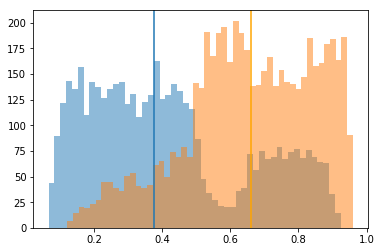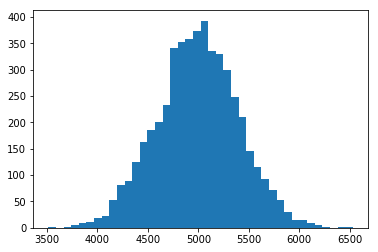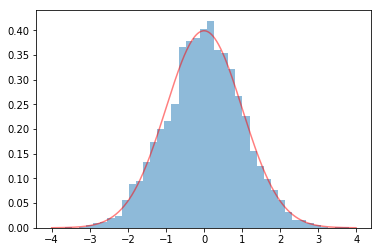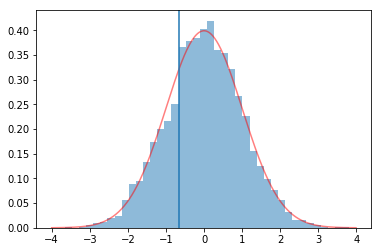# The ROC-AUC and the Mann-Whitney U-test (Wilcoxon rank sum test)

A deepdive into the statistical foundation of the ROC-AUC, its relation to the Mann-Whitney U and Wilcoxon test and its statistical distribution.

The Receiver-Operating-Characteristic-Curve (ROC) and the area-under-the-ROC-curve (AUC) are popular measures to compare the performance of different models in machine learning.

The AUC is a rank measure, which means it abstracts from the differences in the probability scores and only looks at too which extend the target observations are ranked above non-target observations. We can understand the AUC and its properties much better by understanding its relation to the Mann-Whitney U test a.k.a Wilcoxon rank sum test. In particular, we will get a better intuition why the score distribution plays a role for model comparison using the AUC.

The post has the structure:

• Introduction of the ROC-AUC
• The AUC as a rank-sum test
• The Normal distribution of the AUC statistic
• Confidence intervals for the AUC

For an advanced discussion of perfomance metrics including the AUC, see
Hernández-Orallo, J., Flach, P., & Ferri, C. (2012). A unified view of performance metrics: translating threshold choice into expected classification loss. Journal of Machine Learning Research, 13, 2813-2869.

## The ROC-AUC¶

Let's see how the ROC is usually used for model comparison.

In :
import numpy as np
import scipy as sp
import pandas as pd
import matplotlib.pyplot as plt


Generate some sandbox data from a logistic regression model. We include some an additional quadratic effect of the same $X$ to avoid a perfect model.

In :
def generate_data(n, beta_0, beta, sigma):
if hasattr(beta, '__len__'):
X = np.random.uniform(-5,5,size=[n, len(beta)])
else:
X = np.random.uniform(-5,5,size=[n,])
#y = beta_0 + np.dot(X, beta) + np.random.normal(0, sigma, size=[n,])
y = np.random.binomial(n=1, p = 1/(1 + np.exp(-(beta_0 + np.dot(X, beta)
+ 0.4*np.dot(X**2, beta)))))

return X, y

In :
X, y = generate_data(10000, 1, [2,-2], 1)

In :
print(y[0:5])
print(X[0:3])

[0 1 1 1 1]
[[-4.34442399 -4.20340974]
[ 1.68772074 -3.38655471]
[ 2.42107746 -4.33697666]]


Fit a model to the sandbox data to get probability scores. The underlying process has quadratic effects which we ignore in the model, so it will not fit the data perfectly.

In :
from sklearn.linear_model import LogisticRegression

In :
logit = LogisticRegression()
logit.fit(X, y)
print(logit.coef_)

[[ 0.26394901 -0.32417447]]

In :
logit_score = logit.predict_proba(X)[:,1]


Calculate the AUC score given the model predictions.

In :
from sklearn.metrics import roc_auc_score

In :
roc_auc_score(y, logit_score)

Out:
0.7797235340784149

The ROC curve is defined as the sensitivity of the model at each level of (1-specificity) for each probability threshold $t$. Since sensitivity and specificity lie between 0 and 1, the total area between them is 1 and consequently, the area-under-the-ROC-curve lies between 0 and 1.

Why would we do we need the ROC-AUC anyway? We calculate the AUC for different models and pick the model with the highest AUC. Doesn't that like a case for statistical testing?

### The AUC is ranking metric¶

A straight-forward way to show that the ROC/AUC only depends on the ranking of the observations and not on the actual scores is to calculate it on scores that are not bounded between 0 and 1.

In :
# Transform the logit model scores
logit_score2 = np.log(logit_score)*2

In :
np.percentile(logit_score2, [0,0.25,0.5,0.75,1])

Out:
array([-5.42158057, -5.05613808, -4.90290963, -4.80933799, -4.73254133])

These negative values are clearly not probabilities. But they can still be used to rank the observations in the test set. In medicine, blood test levels or similar medical scores can similarly be evaluated for classification using the ROC, so a lot of research on the ROC comes from medicine or biostatistics.

In :
roc_auc_score(y, logit_score2)

Out:
0.7797235340784149

We see that linearly transforming the scores (changing the numbers, but keeping the order the same) does not change the AUC.

## The AUC and the Wilcoxon/Mann-Whitney test¶

The AUC is equivalent to the Wilcoxon or Mann-Whitney U test statistic with the relation:

$AUC = U / (n_0*n_1)$

where $n_1$ and $n_0$ are the number of observations in the target and non-target group, respectively. See https://rmets.onlinelibrary.wiley.com/doi/epdf/10.1256/003590002320603584 for a proof.

The Mann-Whitney U test is used to determine if two independent samples were selected from populations having the same mean rank. Our samples are the model scores for the non-target group and the target group. The mean rank, and so the AUC, can differ with the location of the distribution but also with its shape.

If but only if the distributions are identically shaped but shifted, the U test can be used to determine if there is a difference in medians. See https://www.ncbi.nlm.nih.gov/pmc/articles/PMC1120984/

In :
# Plot score distributions
plt.hist(logit_score[y==0], bins=50, alpha=0.5)
plt.hist(logit_score[y==1], bins=50, alpha=0.5)
# Plot median
plt.axvline(x= np.median(logit_score[y==0]))
plt.axvline(x= np.median(logit_score[y==1]), color="orange")
plt.show()The score distributions in this case are very clearly not the same, they look like they are mirrored. The difference in mean rank is consequently not equivalent to the difference in mean or median. In the original description by Mann and Whitney, they devise the test (in my words) to check if the scores for the target group are stochastically greater than the scores for the non-target group. That means we check if the probability that the test score is above a threshold is always greater for the target than for the non-target group.

So what does the test evaluate? From the formula of the U statistic, we can see that the part that changes from model to model is the sum of the ranks of the observations in the target group.

Let's see how we can calculate the AUC from the U test as an alternative to constructing the ROC curve and calculating its area. Note that we can calculate a U statistic for each of the classes.

vvv The core insight is here vvv

In :
def calc_U(y_true, y_score):
n1 = np.sum(y_true==1)
n0 = len(y_score)-n1

## Calculate the rank for each observation
# Get the order: The index of the score at each rank from 0 to n
order = np.argsort(y_score)
# Get the rank: The rank of each score at the indices from 0 to n
rank = np.argsort(order)
# Python starts at 0, but statistical ranks at 1, so add 1 to every rank
rank += 1

# If the rank for target observations is higher than expected for a random model,
# then a possible reason could be that our model ranks target observations higher
U1 = np.sum(rank[y_true == 1]) - n1*(n1+1)/2
U0 = np.sum(rank[y_true == 0]) - n0*(n0+1)/2

# Formula for the relation between AUC and the U statistic
AUC1 = U1/ (n1*n0)
AUC0 = U0/ (n1*n0)

return U1, AUC1, U0, AUC0


Calculate the U and AUC for the model. Suprise! It's the AUC calculated from the ROC!

In :
calc_U(y, logit_score)

Out:
(19314934.0, 0.7797235340784149, 5456582.0, 0.2202764659215851)
In :
# See why many packages flip model predictions if AUC < 0.5?
# Flipping the output classes flips the reported AUC of class 1.
calc_U(y, 1-logit_score)

Out:
(5456582.0, 0.2202764659215851, 19314934.0, 0.7797235340784149)

Aside: The U statistic does not depend on the ratio between $n_0$ and $n_1$ (since $U_c$ depends only on $n_c$). That's the reason the AUC is not impacted by class imbalance.

## The distribution of the AUC statistic¶

In order to understand the distribution of the AUC, we can make use of our knowledge about the Mann-Whitney U. Unfortunately, I couldn't find a lot of good information out there on the Mann-Whitney or Wilcoxon rank sum test. I find even the original papers short but not very clear.

Be careful with the general guideline to look at the sample with smaller U rather than fix the interesting group in advance. For the AUC correspondance to work as expected, the U statistic should be calculated for the group with higher scores.
The example below shows that the p-value for the Mann-Whitney U test is identical, independent of on which group we calculate the U statistic. Note that I place the mean and median of the normal distribution and t-distribution both at 2 so the test is really identifying a difference in the distribution rather than the mean.

In :
# Make two distributions that are different but similar
# The logit scores from above are so different that the p-value goes to 0
distr1 = sp.random.normal(2,1, size=100)
distr2 = sp.random.standard_t(1, size=100)+2

In :
scipy_U = sp.stats.mannwhitneyu(distr1, distr2, alternative="two-sided", use_continuity=False)
print(scipy_U.statistic)
print(scipy_U.pvalue)

4734.0
0.5157300462863164

In :
scipy_U = sp.stats.mannwhitneyu(distr2, distr1, alternative="two-sided", use_continuity=False)
print(scipy_U.statistic)
print(scipy_U.pvalue)

5266.0
0.5157300462863164


Why is this the case? Because we compare the U statistic observed for either group with the theoretical distribution of the U statistic of a group of that size.
But the sum of ranks clearly also depends on what the highest possible rank is, i.e. on the total number of observations! To make them comparable over different numbers of total observations, the U value is standardized using the mean and standard deviation, which depend on the total number of observations.

In :
# Possible ranks from 0 to n1+n2=200
x = (np.arange(0,200)+1).tolist()

In :
# Under the H_0 that the scores are equally distributed
# Randomly sample ranks and calculate U for n_iter trials
import random

n_iter = 5000 # How fine grained our simulation should be
sim_U = np.empty([n_iter])

# Draw U values under the H0 assumption of random ranks,
# e.g. from a model giving random scores
for it in range(n_iter):
n = 100 # number of samples/group size
s = random.sample(x, n)
ranksum = np.sum(s) - n*(n+1)/2
sim_U[it] = ranksum


In :
# Plot the distribution of the U statistic
import matplotlib.pyplot as plt
plt.hist(sim_U, bins= 40)
plt.show()The distribution of the U statistic under the null hypothesis very quickly approaches the Gaussian (normal) distribution, already at group sizes (for the AUC: observations) of ~20.

In :
# Plot standard normal
plt.plot(np.arange(-4,4,0.01), sp.stats.norm.pdf(x=np.arange(-4,4,0.01),loc=0,scale=1), color='red', alpha=0.5)
# Plot standardized U statistics from simulation
# We should standardize by the theoretical mean and std, but the empirical approximation is enough for show
plt.hist((sim_U - np.mean(sim_U))/np.std(sim_U),  # Standardized U statistics
bins=40, density=True, alpha=0.5)
plt.show()## The AUC as a statistical test¶

Back to our example: We calculate the U statistic for our empirical observations with the goal to show that they are not from a model assigning random scores. The idea for the next steps is the following: We know from our simulation which values of U we expect if the scores are from the same distribution. If our observed U is very different from the expected U, then we can conclude that the scores are not from the same distribution. To make the U easier to compare, we normalize them to look like the second plot above with mean=0 and sd=1

In :
n = len(distr1)
scores = np.concatenate([distr1, distr2])
order = np.argsort(scores)
rank = np.argsort(order)
rank += 1
U_distr1 = np.sum(rank[0:n]) - n*(n+1)/2
U_distr1_normalized = (U_distr1 - 100*100/2) / np.sqrt(100*100*(100+100+1)/12)

print(U_distr1)
print(U_distr1_normalized)

4734.0
-0.6499414439755438


We can calculate the p-value as the probability of finding a more extreme value under our expectation that the U is approximately Gaussian normal distributed. We take the absolute of the value so that we will only have to look on the positive right side. We can do this, because the normal distribution is symmetric. The cumulative distribution function gives us the probability of observing a value $x$ below $z$, i.e. $P(x \leq z)$. We can turn this around as $1 - P(x \leq z)$ to get the probability of seeing a larger value.

For the two-sided test, since the normal distribution is symmetric, the probability of finding a more extreme value on the negative side is exactly the same, leaving us with $2*(1 - P(x \leq z))$.

In :
# Calculate the p-value
# better to use stats.norm.sf() as exact 1-cdf
print("One-sided:", 1 - sp.stats.norm.cdf(abs(U_distr1_normalized)))
print("Two-sided:", 2*(1 - sp.stats.norm.cdf(abs(U_distr1_normalized))))

One-sided: 0.2578650231431582
Two-sided: 0.5157300462863164

In :
# As above:
# Plot standard normal
plt.plot(np.arange(-4,4,0.01), sp.stats.norm.pdf(x=np.arange(-4,4,0.01),loc=0,scale=1), color='red', alpha=0.5)
# Plot standardized U statistics from simulation
plt.hist((sim_U - np.mean(sim_U))/np.std(sim_U),  # Standardized U statistics
bins=40, density=True, alpha=0.5)

# Plot U of our (good) classifier
plt.axvline(U_distr1_normalized)
plt.show()In this case, the U is well within the region that we expect (close to 0), so we wouldn't reject the null hypothesis that the scores come from the same distribution. For a binary model, e.g. the logistic regression above, we can usually be confident that the scores are different for each class, so the p-value in comparison to the random model is usually not helpful.

## A note on confidence intervals of the AUC¶

We can use the approximate variance to calculate confidence intervals for the AUC. This is the correct intuition, but ideally we would use the exact variance, since our approximation may lead to wrong confidence intervals.

Unfortunately, the exact variance only be calculated based on the distributions of the scores (Cortes & Mohri 2005), which typically do not follow a known distribution. There seem to be different suggestions on how to calculate a useful confidence interval, see Cortes & Mohri (2005) for a summary and their (long) formula in Corollary 1 ():

\begin{align} \sigma^2(AUC) &= \frac{(m+n+1)(m+n)(m+n−1)T((m+n−2)Z_4−(2m−n+3k−10)Z_3)} {72m^2n^2} \\ &+ \frac{(m+n+1)(m+n)T(m^2−nm+3km−5m+2k^2−nk+12−9k)Z_2}{48m^2n^2} \\ &− \frac{(m+n+1)^2(m−n)^4 Z_1^2} { 16m^2n^2} − \frac{(m+n+1)Q_1 Z_1}{72m^2n^2} +\frac{kQ_0}{144m^2n^2} \end{align}

with:

\begin{alignat}{2} T &= && 3((m − n)^2 + m + n) + 2 \\ \\ Z_i &= && \frac{ \sum^{k−i}_{x=0} \left(\begin{smallmatrix} m+n+1−i \\ x \end{smallmatrix}\right) } { \sum^k_{x=0} \left(\begin{smallmatrix} m+n+1 \\ x \end{smallmatrix}\right) }\\ \\ Q_0 &= &&(m + n + 1)T k^2 + ((−3n^2 + 3mn + 3m + 1)T − 12(3mn + m + n) − 8)k +\\ & && (−3m^2 +7m + 10n + 3nm + 10)T − 4(3mn + m + n + 1) \\ \\ Q_1 &= && T k^3 + 3(m − 1)T k^2 + ((−3n^2 + 3mn − 3m + 8)T − 6(6mn + m + n))k +\\ & && (−3m^2 +7(m + n) + 3mn)T − 2(6mn + m + n) \end{alignat}

References

Cortes, C., & Mohri, M. (2005). Confidence intervals for the area under the ROC curve. In Advances in neural information processing systems (pp. 305-312). https://cs.nyu.edu/~mohri/pub/area.pdf